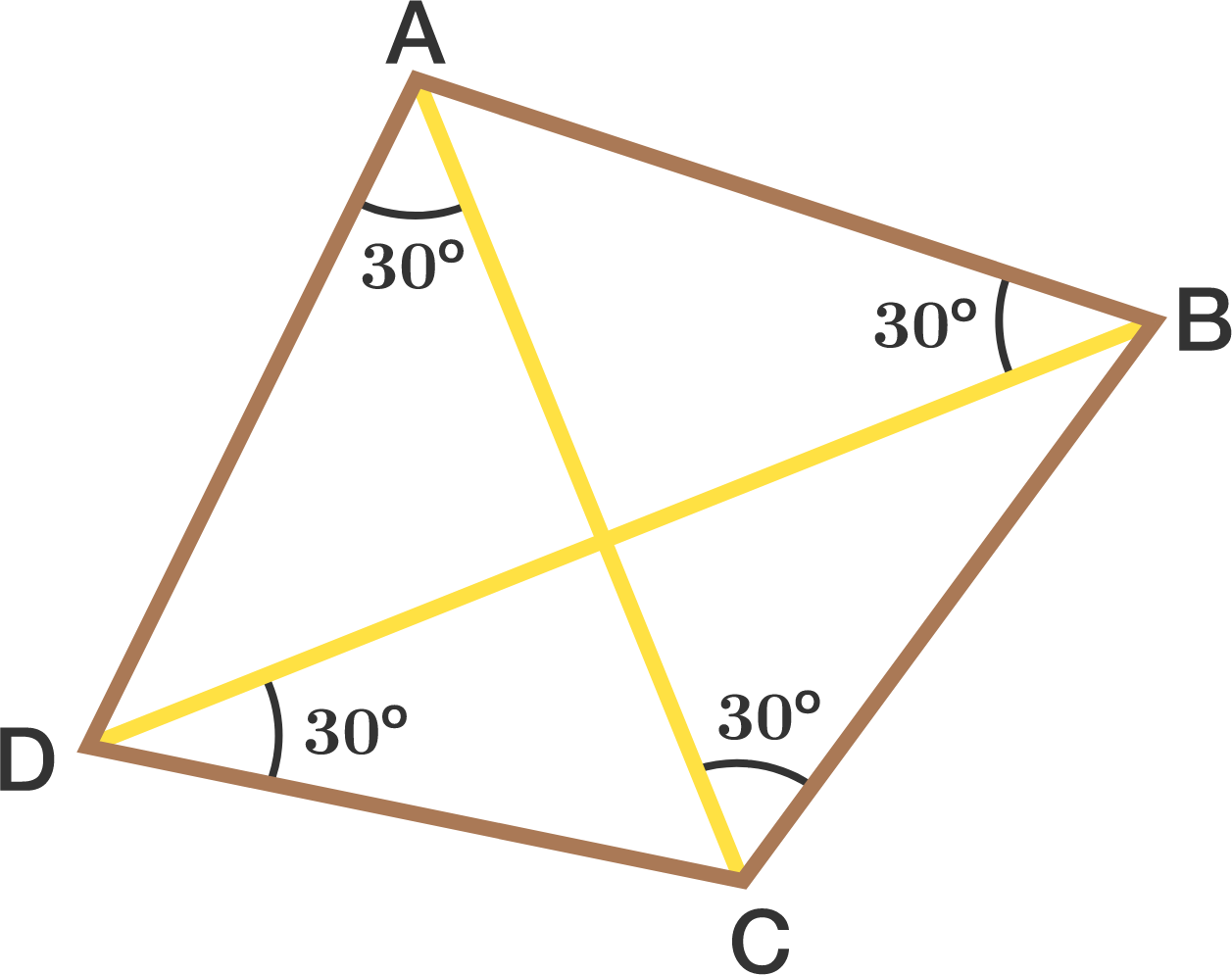# Pinwheel Pattern

Geometry Level 3In a quadrilateral $ABCD$, $\angle ABD = \angle BCA = \angle CDB = \angle DAC = 30^\circ$.

Find the smaller of the two angles (in degrees) between the diagonals AC and BD.


Note: The image might not be necessarily up to scale.

×